# Book/Datasets

(Difference between revisions)
 Revision as of 14:23, 27 October 2009 (view source)Eranders (Talk | contribs)m ← Older edit Current revision as of 15:13, 27 October 2009 (view source)Eranders (Talk | contribs) Line 1: Line 1: = Datasets and Descriptions = = Datasets and Descriptions = - This page is dedicated to datasets that are acceptable for use in the Book.  As many of these data are extremely large, I have not posted them here, instead, I will make sure to go through and put some images next to each description to give an idea of the overall structure of each dataset. + This page is dedicated to datasets that are acceptable for use in the Book.  As many of these data are extremely large, I have not posted them here, instead, I have included links to the download locations.  Additionally, this [http://www.sci.utah.edu/~eranders/data/book_data.vt .vt file] contains versions that will download the appropriate dataset and reproduce the images on this page.  All datasets are included in this .vt except for the .off files. == Synthetic Datasets == == Synthetic Datasets == Line 9: Line 9: | | ==Three Spheres== ==Three Spheres== - * Regular Grid in .raw/.nrrd format.  [http://www.sci.utah.edu/~eranders/data/3big.zip] + * Regular Grid in .raw/.nrrd format.  [http://www.sci.utah.edu/~eranders/data/3big.zip Spheres.zip] ** Datatype  = Float ** Datatype  = Float ** Dimensions = 256 256 256 ** Dimensions = 256 256 256 Line 17: Line 17: | | == Three Spheres with Gaussian Noise == == Three Spheres with Gaussian Noise == - * Regular Grid in .raw/.nrrd format.  [http://www.sci.utah.edu/~eranders/data/3big_noise.zip] + * Regular Grid in .raw/.nrrd format.  [http://www.sci.utah.edu/~eranders/data/3big_noise.zip Noisy_spheres.zip] ** Datatype  = Float ** Datatype  = Float ** Dimensions = 256 256 256 ** Dimensions = 256 256 256 Line 24: Line 24: == Standard Datasets == == Standard Datasets == - + {|{{Prettytable}} - === Fighter === + |[[Image:Fighter.png|256px]] - + | - * Unstructured Tet-Mesh in .off format. + == Fighter == - + * Unstructured Tet-Mesh in .off format. [http://www.sci.utah.edu/~eranders/data/fighter.off Fighter.off] - === Earthquake === + ** Datatype  = Float - + ** Num Vertex = 256,614 - * Unstructured Tet-Mesh in .off format. + ** Num Tets  = 1,403,504 - + ** File Size  = 48 MB - === SPX === + |- - + |[[Image:Spx.png|256px]] - * Unstructured Tet-Mesh in .off format. + | - + == SPX == - === CT-Tooth === + * Unstructured Tet-Mesh in .off format. [http://www.sci.utah.edu/~eranders/data/spx.off Spx.off] - + ** Datatype  = Float - * Regular Grid in .raw/.nrrd format. + ** Num Vertex = 20,108 - + ** Num Tets  = 12,936 - === CT-Head === + ** File Size  = 993 KB - + |- - * Regular Grid in .raw/.nrrd format. + |[[Image:Blunt.png|256px]] + | + == Blunt Fin == + * Unstructured Tet-Mesh in .off format. [http://www.sci.utah.edu/~eranders/data/blunt.off Blunt.off] + ** Datatype  = Float + ** Num Vertex = 40,960 + ** Num Tets  = 187,395 + ** File Size  = 5.8 MB + |- + |[[Image:Tooth.png|256px]] + | + == CT-Tooth == + * Regular Grid in .raw/.nrrd format.   [http://www.sci.utah.edu/~eranders/data/tooth_float.zip Tooth.zip] + ** Datatype  = Float + ** Dimensions = 103, 94, 161 + ** File Size  = 2.0 MB + |} == Collaborator-generated Data == == Collaborator-generated Data == - + {|{{Prettytable}} - === Columbia River === + |[[Image:Cmop.png|256px]] - + | - * Unstructured Prism Mesh converted to .vtk format. + == Columbia River Simulation:  Thanks to Antonio Baptista (STCCMOP) == - + * Unstructured Prism Mesh converted to .vtk format.   [http://www.sci.utah.edu/~eranders/data/columbia_river_002.vtk Inlet.vtk] - === Tokamak Fusion === + ** Num Vertex  = 33,410 - + ** Num Prisms  = 57,825 - * Unstructured Hex Mesh converted to .vtk format. + ** File Size    = 4.4 MB - + ** Scalar Fields - === Cosmology Simulation === + *** salt - salinity field - + *** vpos - vertical position - * Point cloud converted to .vtk format + *** h - bathymetry + *** saltflux - salinity flux + ** Vector Fields + *** uv - horizontal current velocities + |- + |[[Image:Coastal.png|256px]] + | + == Coastal Ocean Simulation:  Thanks to Yvette Spitz (STCCMOP) == + * Curvilinear Grid converted to .vtk format.  [http://www.sci.utah.edu/~eranders/data/0007009.vtk Coastal.vtk] + ** Dimensions = 384, 128, 44 + ** File Size  = 25 MB + ** Scalar Fields + *** phytoplankton - Phytoplankton Concentration + *** zooplankton  - Zooplankton Concentration + *** NO3          - Nitrate Level + *** temperature  - Temperature (Degrees Celsius) + ** Vector Fields + *** velocity      - 3D Water Velocity + |- + |[[Image:Tokamak.png|256px]] + | + == Tokamak Fusion:  Thanks to Allen Sanderson (SDM) == + * Unstructured Hex Mesh converted to .vtk format.   [http://www.sci.utah.edu/~eranders/data/fusion_0067_bin.vtk Tokamak.vtk] + ** Num Vertex = 622,261 + ** Num Hexes  = 600,000 + ** File Size  = 59 MB + ** Scalar Field + *** scalars  -  Temperature (Degrees Kelvin) + ** Vector Field + *** vectors  -  Magnetic Field + |- + |[[Image:Cosmo.png|256px]] + | + == Cosmology Simulation:  Thanks to Katrin Heitmann (LANL) == + * Point cloud converted to .vtk format.  [http://www.sci.utah.edu/~eranders/data/enzo_parts_16.vtk Cosmology.vtk] + ** Num Points = 1,048,576 + ** File Size  = 45 MB + ** Scalar Field + *** mass -  Mass (Solar Masses) + *** tag  -  Id + ** Vector Field + *** velocity - 3D velocity + |- + |[[Image:Mri.png|256px]] + | + == Magnetic Resonance Image:  Thanks to John Schreiner == + * Regular Grid converted to .vtk format.  [http://www.sci.utah.edu/~eranders/data/mri.vtk Mri.vtk] + ** Dimensions = 256, 256, 208 + ** Datatype  = Unsigned Short + ** File Size  = 27 MB + |}

# Datasets and Descriptions

This page is dedicated to datasets that are acceptable for use in the Book. As many of these data are extremely large, I have not posted them here, instead, I have included links to the download locations. Additionally, this .vt file contains versions that will download the appropriate dataset and reproduce the images on this page. All datasets are included in this .vt except for the .off files.

## Synthetic Datasets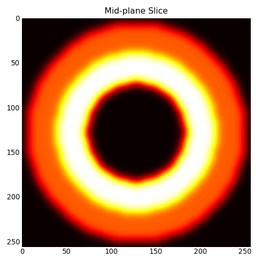## Three Spheres

• Regular Grid in .raw/.nrrd format. Spheres.zip
• Datatype = Float
• Dimensions = 256 256 256
• File Size = 50 MB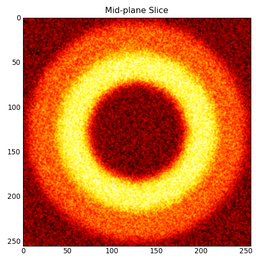## Three Spheres with Gaussian Noise

• Regular Grid in .raw/.nrrd format. Noisy_spheres.zip
• Datatype = Float
• Dimensions = 256 256 256
• File Size = 58 MB

## Standard Datasets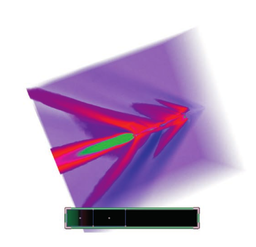## Fighter

• Unstructured Tet-Mesh in .off format. Fighter.off
• Datatype = Float
• Num Vertex = 256,614
• Num Tets = 1,403,504
• File Size = 48 MB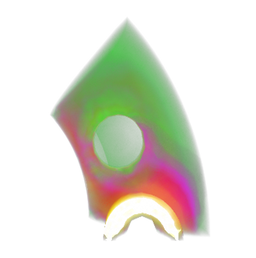## SPX

• Unstructured Tet-Mesh in .off format. Spx.off
• Datatype = Float
• Num Vertex = 20,108
• Num Tets = 12,936
• File Size = 993 KB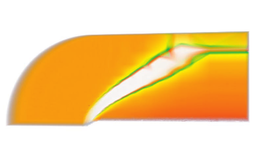## Blunt Fin

• Unstructured Tet-Mesh in .off format. Blunt.off
• Datatype = Float
• Num Vertex = 40,960
• Num Tets = 187,395
• File Size = 5.8 MB## CT-Tooth

• Regular Grid in .raw/.nrrd format. Tooth.zip
• Datatype = Float
• Dimensions = 103, 94, 161
• File Size = 2.0 MB

## Collaborator-generated Data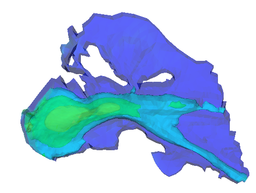## Columbia River Simulation: Thanks to Antonio Baptista (STCCMOP)

• Unstructured Prism Mesh converted to .vtk format. Inlet.vtk
• Num Vertex = 33,410
• Num Prisms = 57,825
• File Size = 4.4 MB
• Scalar Fields
• salt - salinity field
• vpos - vertical position
• h - bathymetry
• saltflux - salinity flux
• Vector Fields
• uv - horizontal current velocities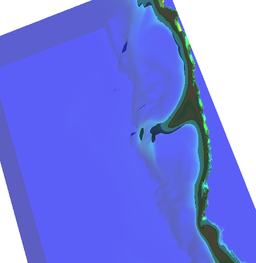## Coastal Ocean Simulation: Thanks to Yvette Spitz (STCCMOP)

• Curvilinear Grid converted to .vtk format. Coastal.vtk
• Dimensions = 384, 128, 44
• File Size = 25 MB
• Scalar Fields
• phytoplankton - Phytoplankton Concentration
• zooplankton - Zooplankton Concentration
• NO3 - Nitrate Level
• temperature - Temperature (Degrees Celsius)
• Vector Fields
• velocity - 3D Water Velocity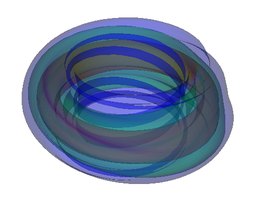## Tokamak Fusion: Thanks to Allen Sanderson (SDM)

• Unstructured Hex Mesh converted to .vtk format. Tokamak.vtk
• Num Vertex = 622,261
• Num Hexes = 600,000
• File Size = 59 MB
• Scalar Field
• scalars - Temperature (Degrees Kelvin)
• Vector Field
• vectors - Magnetic Field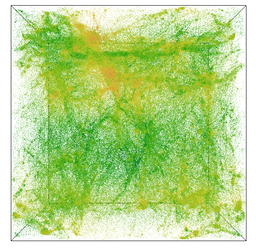## Cosmology Simulation: Thanks to Katrin Heitmann (LANL)

• Point cloud converted to .vtk format. Cosmology.vtk
• Num Points = 1,048,576
• File Size = 45 MB
• Scalar Field
• mass - Mass (Solar Masses)
• tag - Id
• Vector Field
• velocity - 3D velocity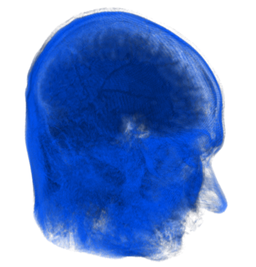## Magnetic Resonance Image: Thanks to John Schreiner

• Regular Grid converted to .vtk format. Mri.vtk
• Dimensions = 256, 256, 208
• Datatype = Unsigned Short
• File Size = 27 MB